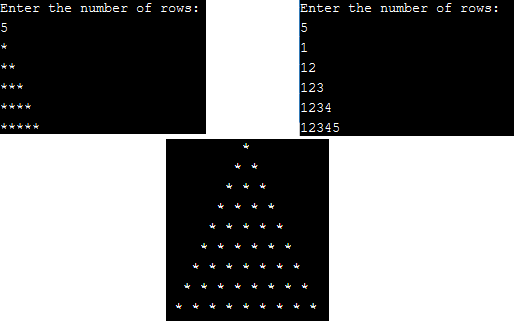If you have just started coding in Java, one of the basic program which every one code while learning about loops is Java Patterns programs based on star pattern or number pattern, so in this article, I have provided some samples of java pyramid pattern programs.### Pyramid pattern using Star

In this code, we will be creating right-angled triangle pattern program using stars.

First, we will ask user to input the number of rows to print using `Scanner` Class.

Once we have total number of rows to be printed, we will use for loop to print * for each row.

``````import java.util.Scanner;
public class Main
{
public static void main(String[] args) {
int i;
int j;
Scanner scan=new Scanner(System.in);

System.out.println("Enter the number of rows: ");
int rows=scan.nextInt(); //Takes input from the user for number of rows

for(i=1;i<=rows;i++)
{
for(j=1;j<=i;j++)
{
System.out.print("*");

}
System.out.println();

}
}
}
``````

Output:

``````Enter the number of rows:
5
*
**
***
****
*****
``````

Basically, when the code executed and we have entered number of rows = 5.

For loop is executed, for first for loop ( outer loop) condition is checked, as it is true, inner for loop is executed.

value of j <= i ( j=1, i =1) for first loop, hence it is true and "*" is printed for first row.

Again, same logic is applied but as value of j= 2 in second loop which is greater than 1, so next outer loop is executed, i.e , i = 2 now.

Now inner loop is executed twice, and so on.

### Pyramid star pattern using Numbers

We will be using same logic as above, but we will replace "*" with numbers of inner loop.

``````import java.util.Scanner;
public class Main
{
public static void main(String[] args) {
int i;
int j;
Scanner scan=new Scanner(System.in);

System.out.println("Enter the number of rows: ");
int rows=scan.nextInt(); //Takes input from the user for number of rows

for(i=1;i<=rows;i++)
{
for(j=1;j<=i;j++)
{
System.out.print(j);

}
System.out.println();

}
}
}
``````

Output:

``````Enter the number of rows:
5

1
12
123
1234
12345``````

You can try it using the editor below

### Equilaternal triangle using Star (*)

``````public class Main
{

public static void main(String [] args) {
int size=10;
int i, j;
//get row size of triangle
for(i=1;i<=size;i++) {
for(j=1;j<=size;j++) {

if((i+j)>size) {  // add row * and space
System.out.print("*");
System.out.print(" ");
}
else    // add row spaces, before printing stars
{
System.out.print(" ");
}
}
System.out.println();
}
}

}
``````

Output:

``````         *
* *
* * *
* * * *
* * * * *
* * * * * *
* * * * * * *
* * * * * * * *
* * * * * * * * *
* * * * * * * * * * ``````

In the above program, suppose first loop is executed and we have i = 1, size =10 and inner loop j=1

So, in the code, first it is checked, if (i+j) = 2 ( for first loop) is greater than total size (10).

If not, print spaces first and if yes, print *.

Similarly, more loops are executed.

You may also like to read:

Top Java Interview questions